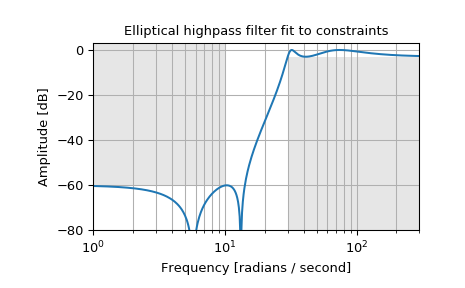# scipy.signal.ellipord¶

scipy.signal.ellipord(wp, ws, gpass, gstop, analog=False)[source]

Elliptic (Cauer) filter order selection.

Return the order of the lowest order digital or analog elliptic filter that loses no more than gpass dB in the passband and has at least gstop dB attenuation in the stopband.

Parameters: wp, ws : float Passband and stopband edge frequencies. For digital filters, these are normalized from 0 to 1, where 1 is the Nyquist frequency, pi radians/sample. (wp and ws are thus in half-cycles / sample.) For example: Lowpass: wp = 0.2, ws = 0.3 Highpass: wp = 0.3, ws = 0.2 Bandpass: wp = [0.2, 0.5], ws = [0.1, 0.6] Bandstop: wp = [0.1, 0.6], ws = [0.2, 0.5] For analog filters, wp and ws are angular frequencies (e.g. rad/s). gpass : float The maximum loss in the passband (dB). gstop : float The minimum attenuation in the stopband (dB). analog : bool, optional When True, return an analog filter, otherwise a digital filter is returned. ord : int The lowest order for an Elliptic (Cauer) filter that meets specs. wn : ndarray or float The Chebyshev natural frequency (the “3dB frequency”) for use with ellip to give filter results.

ellip
Filter design using order and critical points
buttord
Find order and critical points from passband and stopband spec
iirfilter
General filter design using order and critical frequencies
iirdesign
General filter design using passband and stopband spec

Examples

Design an analog highpass filter such that the passband is within 3 dB above 30 rad/s, while rejecting -60 dB at 10 rad/s. Plot its frequency response, showing the passband and stopband constraints in gray.

>>> from scipy import signal
>>> import matplotlib.pyplot as plt

>>> N, Wn = signal.ellipord(30, 10, 3, 60, True)
>>> b, a = signal.ellip(N, 3, 60, Wn, 'high', True)
>>> w, h = signal.freqs(b, a, np.logspace(0, 3, 500))
>>> plt.semilogx(w, 20 * np.log10(abs(h)))
>>> plt.title('Elliptical highpass filter fit to constraints')
>>> plt.ylabel('Amplitude [dB]')
>>> plt.grid(which='both', axis='both')
>>> plt.fill([.1, 10,  10,  .1], [1e4, 1e4, -60, -60], '0.9', lw=0) # stop
>>> plt.fill([30, 30, 1e9, 1e9], [-99,  -3,  -3, -99], '0.9', lw=0) # pass
>>> plt.axis([1, 300, -80, 3])
>>> plt.show()#### Previous topic

scipy.signal.ellip

#### Next topic

scipy.signal.bessel